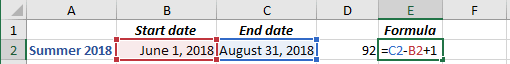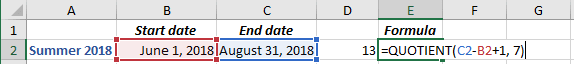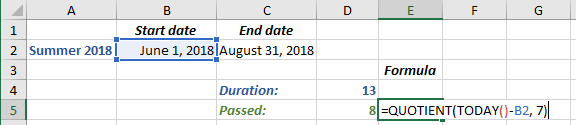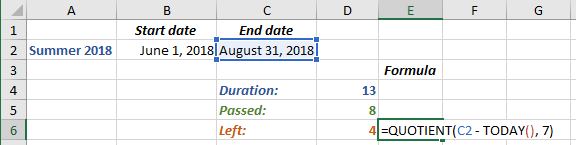# How many weeks left to or passed from the specific date in Excel

Excel
When you plan a trip, project, etc. you can need to calculate a number of days or weeks between the start and end dates, or how many days or weeks left/passed. This tip is about an easy and fast way of complete weeks calculation.

1.   If you need to calculate a number of complete weeks between two dates, do the following (for the calculation of weeks left/passed see below):

1.1.   Calculate the number of days between two dates:

= <end date> - <start date> + 1

For example, to calculate the number of days in summer 2018:See Calculating the Number of Days between two dates that explains why + 1 is added.

1.2.   Divide the number of days by 7 (number of days at one week). It is necessary to return only the integer part of the division result and discard the remainder of the division result, so, use the QUOTIENT function:

= QUOTIENT (numerator, denominator)

where:

• numerator is a dividend, a number to be divided,
• denominator is a divisor, a number to divide by.

For this example:

= QUOTIENT (<end date> - <start date> + 1, 7)The 1st of June 2018 is Friday, and the 31st of August 2018 is Friday. So, the number of complete weeks between these dates is 13.

2.   If you need to calculate a number of complete weeks passed from some date, use the formula:

= QUOTIENT (TODAY () - <start date>, 7)

where TODAY () calculates the current date.

For example, for the 1st of August 2018:3.   If you need to calculate a number of complete weeks left to some date, use the formula:

= QUOTIENT (<end date> - TODAY (), 7)

For example, for the 1st of August 2018:See also this tip in French: Combien de semaines restent ou se sont écoulées à partir de la date spécifique dans Excel.